# DSST Propagation results seems odd

Hello orekit community,

I’m a new user of the orekit python wrapper (I use it for like 5 days). My goal is to propagate Keplerian parameters that I got from a TLE using the DSST propagator. (Tell me if this methodology seems odd to you or not in the first place).
So I began extracting all the data I have from a .txt file containing a lot of TLEs (Topex). Then I only kept the first data of the first TLE and try to integrate using the Runge-Kutta integrator (order 4) and the DSST propagator. I wanted to take only the attraction of the earth and the J2 influence.

Then I propagated and try to get the parameters I wanted and plot them. I propagated every second.

But the results I got seems very odd and wrong.

Here are the .py and the file I used :

Approche_1_DSST.py (10.0 KB)
2256.txt (1.2 MB)

(Put the 2256.txt file into a folder named ‘TLEs’)

Thank you in advance, I hope you will found out what’s wrong, or at least what could be better. Also If my methodology seems odd let me know it.

Hi @JulienL

``````for i in range(len(TLEs)):
tle_i.append(TLEs[i].getI())
tle_omega.append(TLEs[i].getRaan())
tle_e.append(TLEs[i].getE())
tle_arg.append(TLEs[i].getPerigeeArgument())
tle_M.append(TLEs[i].getMeanAnomaly())
tle_date.append(TLEs[i].getDate())
tle_meanMotion.append(TLEs[i].getMeanMotion())
``````

You can’t use directly the orbital parameters contained in the TLE.
These parameters are only significant with respect to the propagation model (e.g. SGP4). Therefore, you must call the `TLEPropagator` to compute the correct orbital elements at the TLE epoch before using them. I’m not a Python expert, but you can try something like that:

``````for i in range(len(TLEs)):
propagator = TLEPropagator.selectExtrapolator(TLEs[i])
orbit = propagator.propagate(TLEs[i].getDate()).getOrbit()
keplerian = OrbitType.KEPLERIAN.convertType(orbit)
tle_i.append(keplerian.getI())
tle_a.append(keplerian.geSma())
...
``````

Other remarks.

• If you only want to consider J2 perturbation, you can remove the following line: `propagator.addForceModel(DSSTZonal(provider))`

• Using DSST propagator, you can increase the integration step of the Runge-Kutta integrator. For instance, for LEO satellites you can try using 5 orbital periods. For MEO/GEO an integration step of 43200 seconds is acceptable.

Best regards,
Bryan

1 Like

So I don’t need to use the initial conditions : e0, i0, pa0, a0, raan0 and M0 ? Also, If I only use the TLEPropagator then I don’t need to use an integrator right ? This would delete a great part of my code where I tried to use the initial conditions to propagate.

I understand your remarks about the Runge-Kutta integrator and I also found 5 orbital periods in the litterature.

I didn’t find any callable function .getSma() but I have .getA() which I suppose is the same. I removed the J2 perturbation.

Moreover, I commented a great part of the .py and added your suggestion (I just wanted to have the initial data still so I kept the tle_ lists and just used the prop_ lists). The results I have are still really strange. I tried to look the len of each and every prop_ list but the size is the same as initial datas.

For example I got this for the inclination propagation :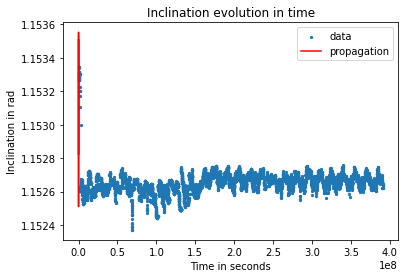It seems like it only propagated in only one step…

Approche_1_DSST.py (12.4 KB)

You need the initial conditions for initialization, but you can’t directly access the initial conditions using the extracted TLE information. That’s why I recommended you to use

``````propagator = TLEPropagator.selectExtrapolator(TLEs[i])
orbit = propagator.propagate(TLEs[i].getDate()).getOrbit()
``````

to access the initial conditions.

SGP4 model is an analytical propagation model taking into account the following perturbations:

• J2 to J5 zonal harmonics
• Sun/Moon attraction
• Drag (based on the B* value)

If you want to evaluate the effect of the J2 perturbation, using SGP4 model you will take into account more effects.
However, the recommended way to extrapolate TLE is the SGP4 modelUsing another model is not recommended.

Yes, my bad. I wrote the code directly in the forum.

``````# Here is the step between each propagation in seconds
step = 1.0  # in sec

for i in range(len(TLEs)):
propagator = TLEPropagator.selectExtrapolator(TLEs[i])
orbit = propagator.propagate(TLEs[i].getDate(), TLEs[i].getDate().shiftedBy(step)).getOrbit()
keplerian = OrbitType.KEPLERIAN.convertType(orbit)
prop_a.append(keplerian.getA())
prop_i.append(keplerian.getI())
prop_e.append(keplerian.getE())
``````

That’s because you propagate for 1 second.
If you want to evaluate the first TLE, you can try to propagate the orbit for a longer time span, to the last TLE epoch for instance, and save the orbital elements for different fixed step using a step handler. You can look at the following example for initializing a step handler: src/main/java/org/orekit/tutorials/propagation/NumericalPropagation.java · master · Orekit / Orekit tutorials · GitLab

It’s in Java and using a numerical propagator but it can be easily adapted.

1 Like

Hi @bcazabonne ,

Thank you for your reply, it helped me a lot and I was able to propagate with the TLEPropagator, and the results I have seems pretty good ! (except for the eccentricity because the SGP4 method is not quite accurate on this one)

I am now trying to use the DSST Propagator, which is quite useful, but needs forceModels to be added.
So I added the newton attraction with :

central_force = DSSTNewtonianAttraction(mu)

I also added two other bodies to the propagation, the sun and the moon :

sun = CelestialBodyFactory.getSun()
moon = CelestialBodyFactory.getMoon()

And finally the DSSTZonal (and therefore I founded the documentation about this one, I am still not sure of what It does. It’s may be the center of my current problem) :

unnormal_provider = GravityFieldFactory.getUnnormalizedProvider(10,10)

Then I propagate using as initial condition the least square approximation of the first keplerian parameters of my TLE file.
The TLE file used : (ISS) Zarya , last month :
2256.txt (1.4 MB)

I plot all the data I gathered through propagation and the initial data :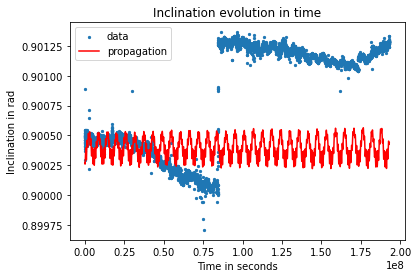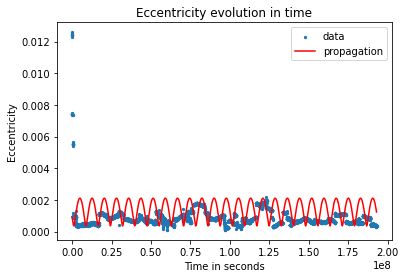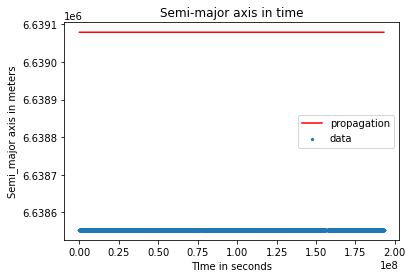I am not sure what’s wrong here, the forces added to the DSST model seems good and work without any problem. But still the results are really really different from the initial data.

Here is the .py :
Approche_1_DSST.py (13.7 KB)

Hey there,

In Orekit, if you have defined the initial conditions with an `Orbit` object within the `SpacecraftState`, propagators automatically add the keplerian force upon creation so you do not need to add it.

Best,
Romain

1 Like

Okay ! I didn’t know, thank you @Serrof ! I removed it but the graphs still looks the same.

Hi @JulienL,

You have a problem here, the second argument of `DSSTThirdBody` should be the central attraction coefficient, so Earth µ and not the µ of the Moon or the Sun.
I reckon it’s not very natural to do so but the central µ is needed to compute some auxiliary quantities in `DSSTThirdBodyContext`

1 Like

Yes… we shall improve this JavaDoc.

1 Like

I agreeAlthough the Javadoc is technically correct we should probably make it clearer with bold fonts or a warning or a lengthier explanation because it’s very counter-intuitive.

1 Like

The magic of DSSTHi all,

Thank you for you replies, It helped me a lot. Yeah it didn’t seems really intuitive at first glance. I’m going to open an issue then : DSSTThirdBody Java doc warning about the µ constant to use (#1057) · Issues · Orekit / Orekit · GitLab

Here are the results I got when changing the µ of bodies with the µ of earth :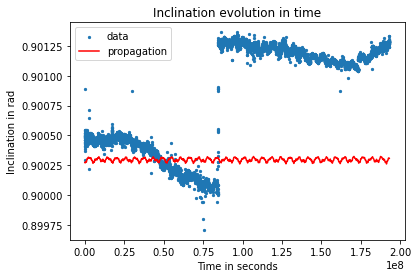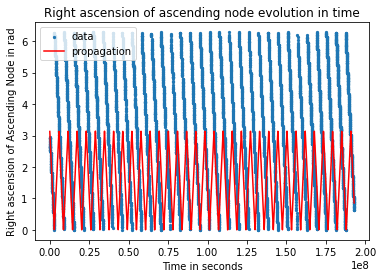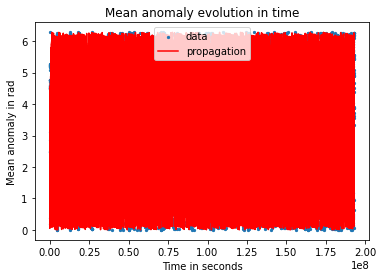Results seems better, still I have an offset for the semi-major axis, and an offset of π for the raan. Do you have an explanation for that ?

Unfortunately, I can’t open the .py file with my phone… So, I ask you the question. How do you compute the `data` values? Are they the result of the TLE propagation?
If yes, I think you are comparing osculating parameters (from the TLE propagation) with mean parameters (from DSST propagation). That’s probably the reason why you have offsets.
For the RAAN, it looks like a difference of reference for angle normalization.

Bryan

1 Like

Hi @bcazabonne ,

In fact the `data` plotted are the raw data inside the TLE file. I created a function that extracts a list of TLEs objects containing all the raw data of the file. Then from each TLE object I extract the keplerian parameters that I plot.

Then, for the propagation, I only use the first keplerian parameters of the first TLE as an initial condition to propagate.

The model that I use here with the `DSSTPropagator` uses the attraction of the earth (initially contained in the DSST method like @Serrof said) the attraction of the sun and the Moon, and the solar radiation pressure.

Finally I propagate with the `DSSTPropagator` with a number of occurrences and a step that fit the number of TLE and the duration from the first to the last date of the file.

I didn’t think about the angle normalization for the raan, I will take a closer look to that, thank you.

I tried to setup an atmospheric drag but I still can’t manage to find a way to make it works.